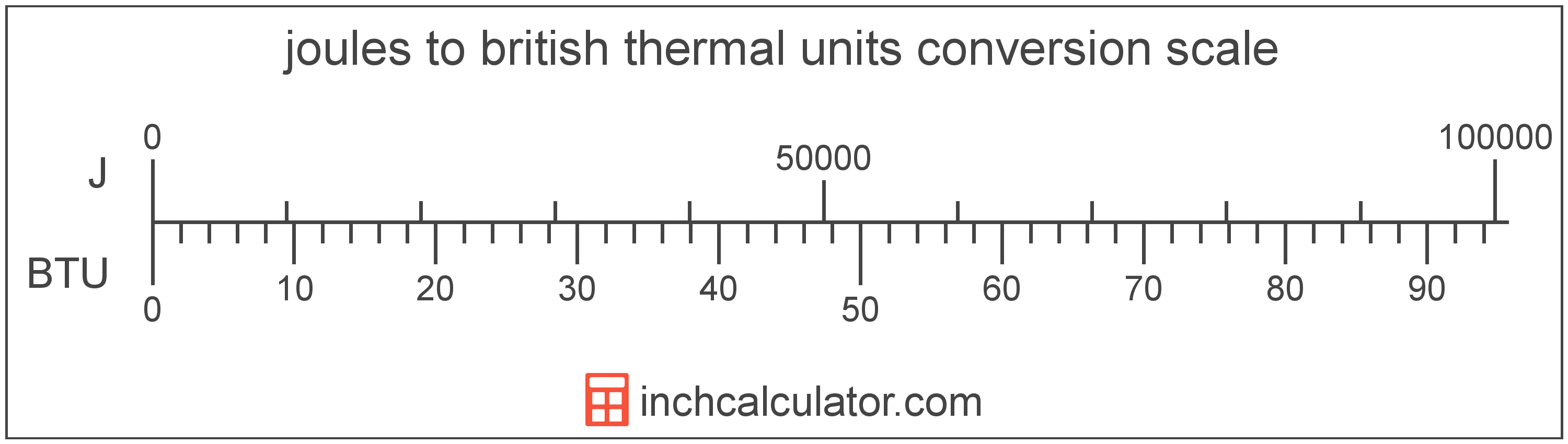# Joules to British Thermal Units Conversion

Enter the energy in joules below to get the value converted to british thermal units.

Results in British Thermal Units:1 J = 0.000948 BTU

## How to Convert Joules to British Thermal Units

To convert a joule measurement to a british thermal unit measurement, multiply the energy by the conversion ratio.

Since one joule is equal to 0.000948 british thermal units, you can use this simple formula to convert:

british thermal units = joules × 0.000948

The energy in british thermal units is equal to the joules multiplied by 0.000948.

For example, here's how to convert 5,000 joules to british thermal units using the formula above.
5,000 J = (5,000 × 0.000948) = 4.739086 BTU## Joules

The joule is the energy equal to the force on an object of one newton at a distance of one meter. One joule is equal to the heat energy dissipated by the current of one ampere through one ohm of resistance for one second.

One joule is also equal to the energy needed to move an electric charge of one coulomb through a potential difference of one volt. In addition, one joule is also equal to the one watt-second.

The joule is the SI derived unit for energy in the metric system. Joules can be abbreviated as J; for example, 1 joule can be written as 1 J.

## British Thermal Units

British thermal units are a measure of heat energy. Specifically, one british thermal unit is equal to the amount of heat energy required to increase the temperature of one pound of water one degree Fahrenheit. They are often used as a way to rate the energy heat producing appliances such furnaces, and to price heating fuels such as natural gas.

The british thermal unit is a US customary unit of energy. British thermal units can be abbreviated as BTU; for example, 1 british thermal unit can be written as 1 BTU.

## Joule to British Thermal Unit Conversion Table

Joule measurements converted to british thermal units
Joules British Thermal Units
1 J 0.000948 BTU
2 J 0.001896 BTU
3 J 0.002843 BTU
4 J 0.003791 BTU
5 J 0.004739 BTU
6 J 0.005687 BTU
7 J 0.006635 BTU
8 J 0.007583 BTU
9 J 0.00853 BTU
10 J 0.009478 BTU
20 J 0.018956 BTU
30 J 0.028435 BTU
40 J 0.037913 BTU
50 J 0.047391 BTU
60 J 0.056869 BTU
70 J 0.066347 BTU
80 J 0.075825 BTU
90 J 0.085304 BTU
100 J 0.094782 BTU
200 J 0.189563 BTU
300 J 0.284345 BTU
400 J 0.379127 BTU
500 J 0.473909 BTU
600 J 0.56869 BTU
700 J 0.663472 BTU
800 J 0.758254 BTU
900 J 0.853035 BTU
1,000 J 0.947817 BTU
2,000 J 1.8956 BTU
3,000 J 2.8435 BTU
4,000 J 3.7913 BTU
5,000 J 4.7391 BTU
6,000 J 5.6869 BTU
7,000 J 6.6347 BTU
8,000 J 7.5825 BTU
9,000 J 8.5304 BTU
10,000 J 9.4782 BTU

## References

1. International Bureau of Weights and Measures, The International System of Units, 9th Edition, 2019, https://www.bipm.org/documents/20126/41483022/SI-Brochure-9-EN.pdf